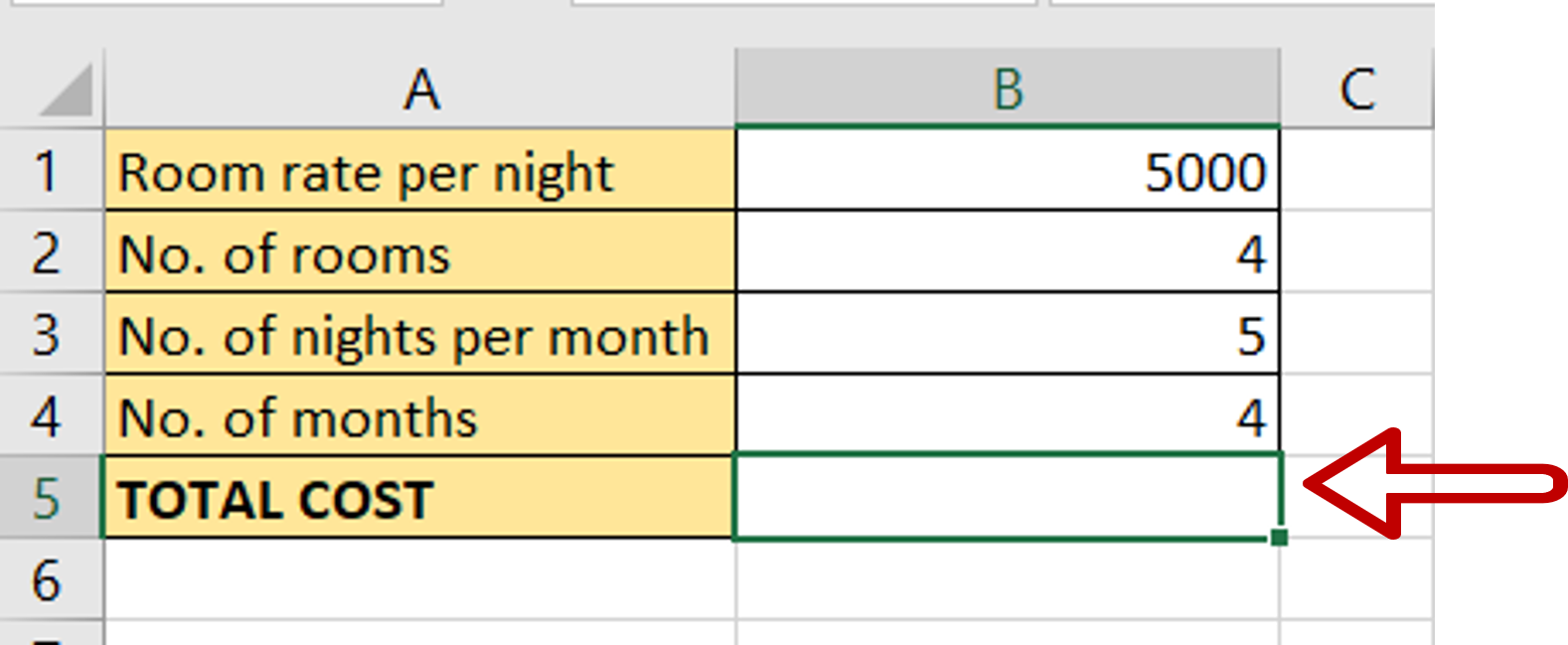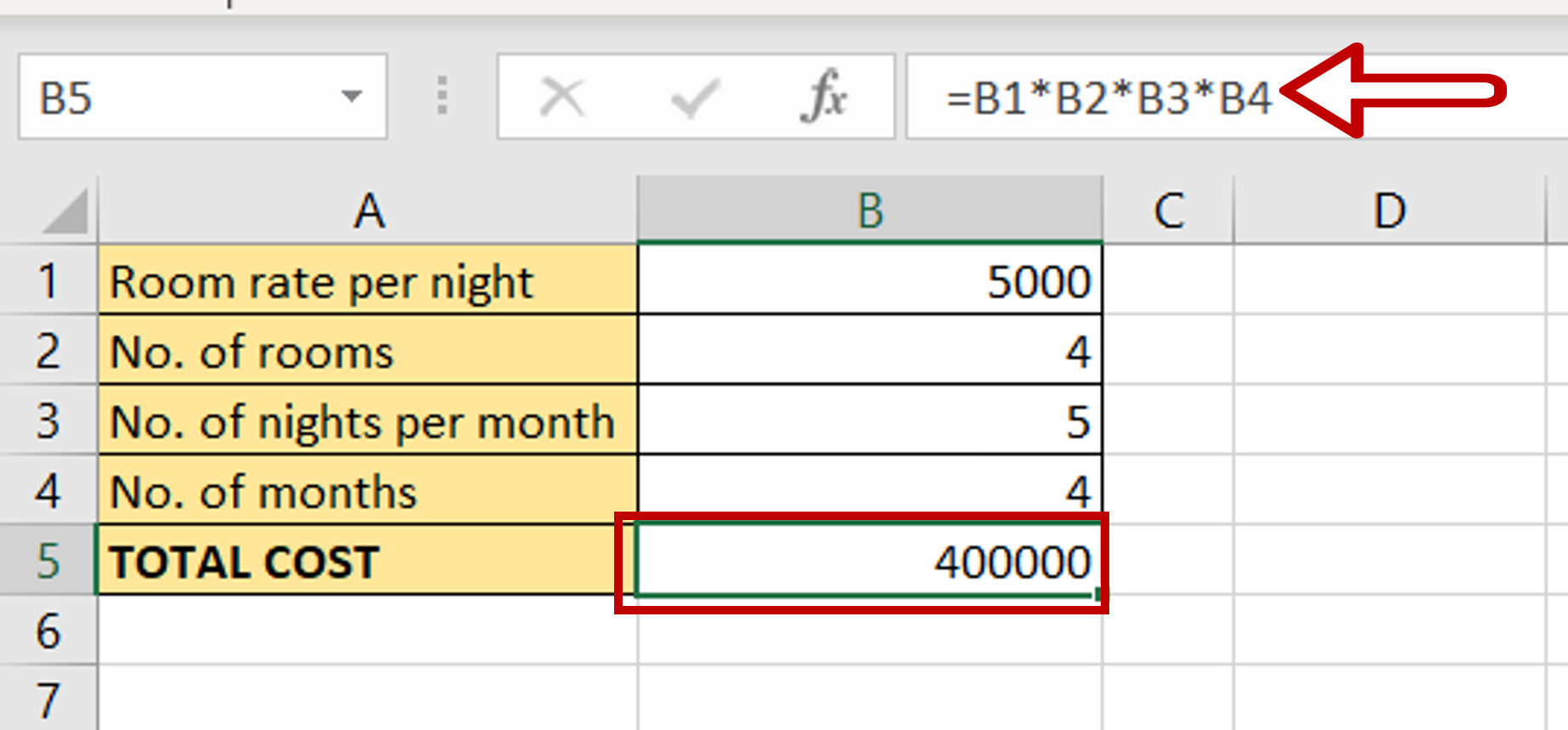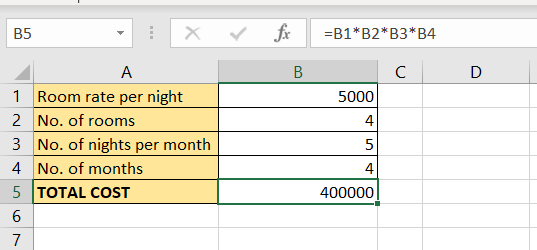# How do you multiply cells in Excel

You can watch a video tutorial here.Excel is widely used for calculations due to the several arithmetic operators and functions that it has. Multiplication is one of the basic mathematical operations and when using Excel for calculations, there will be many occasions where you will need to multiply cells. In Excel, you can use cell references instead of the actual numbers so that the formula is dynamic and the result is updated each time the cell value changes.

### Step 1 – Select the destination– Select the cell in which the result is to be displayed

### Step 2 – Multiply the cells– Type the formula using cell references:
= Room rate per night * No. of rooms * No. of nights per month * No. of months
– Press Enter

### Step 3 – Check the result– The cells are multiplied and the result is displayed in the destination cell